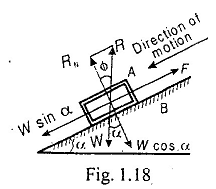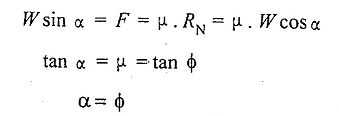# Angle of Repose

Angle of Repose - Where the angle of inclination (α) of the plane is equal to the angle of friction (ϕ).It is the angle of inclination (α) of the plane to the horizontal, at which the body just begins to move down the plane. A little consideration will show that the body will begin to move down the plane, if the angle of inclination (α) of the plane is equal to the angle of friction (ϕ). From Fig. 1.18, we find that